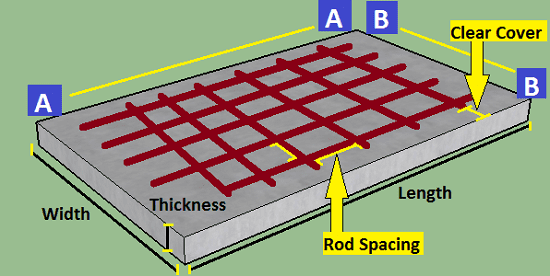# Slab Estimate Calculator | Details of Steel and Concrete

Slab Estimate Calculator calculates the volume of sand, the weight of cement, weight of steel required to construct a cubical slab.

## Slab Estimate Calculator## How to use this tool?

#### Standard Data used in this Calculator

• Added 54% extra mortar to convert wet volume into dry volume.
• Density of cement = 1440 Kg/m3
• Density of Steel Rod = 7850 Kg/m3
• 1 meter = 3.28084 feet
• 1 bags of cement = 50 Kg

## Some other Calculator

Steel Pipe Weight Calculator with total Cost

Building Cost Estimator/Calculator

Irregular land Area Calculator in Sq. Feet and Sq. Meter

Sharing Is Caring: